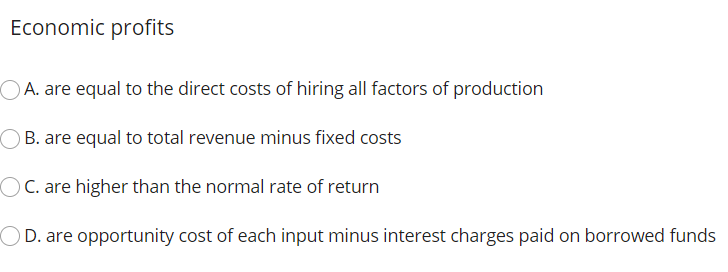### Create an Account

Home / Questions / Economic profits A. are equal to the direct costs of hiring all factors of production OB. ...

# Economic profits A. are equal to the direct costs of hiring all factors of production OB. are equal to total revenue minus fixed costs C. are higher than the normal rate of return D. are opportunity c

Economic profits A. are equal to the direct costs of hiring all factors of production OB. are equal to total revenue minus fixed costs C. are higher than the normal rate of return D. are opportunity cost of each input minus interest charges paid on borrowed fundsApr 20 2021 View more View LessSubscribe To Get Solution